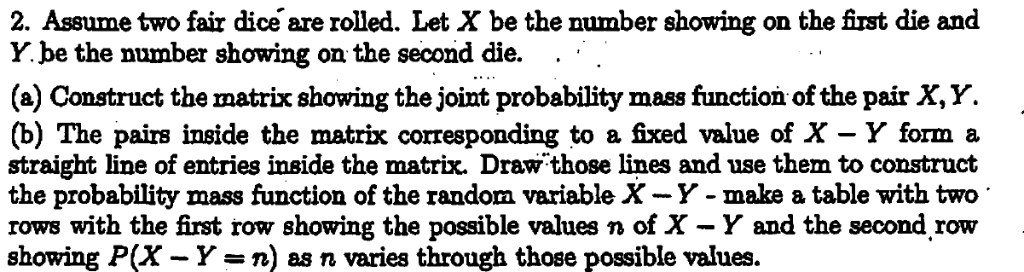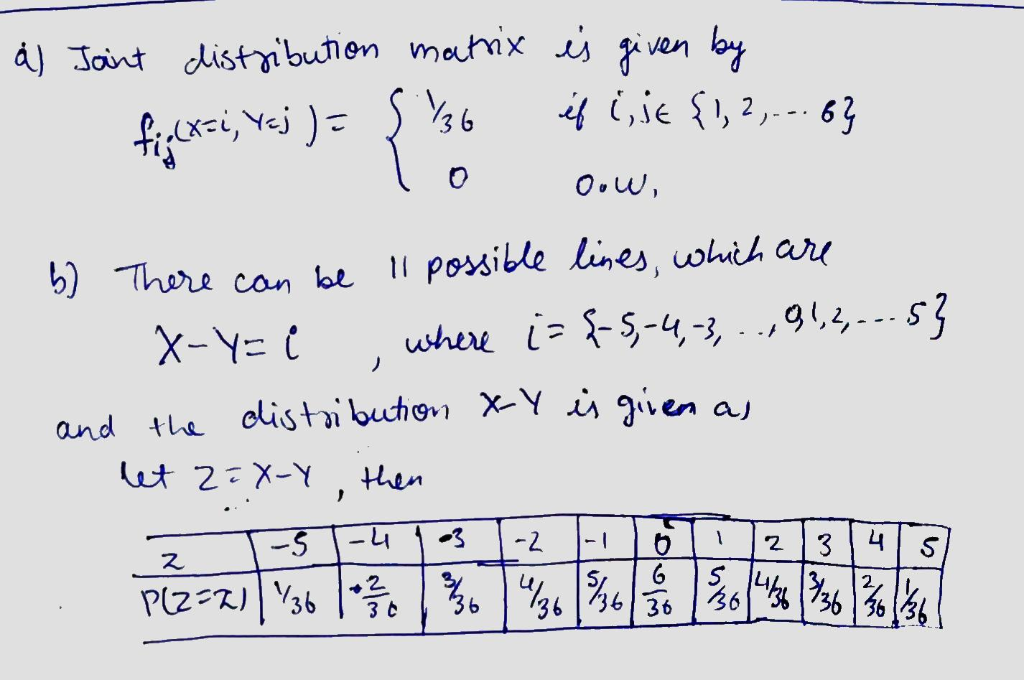# 2. Assume two fair dice are rolled. Let X be the number showing on the first die and number showing on the second die. (a) Construct the matrix showing the joint probability mass function of the pair...2. Assume two fair dice are rolled. Let X be the number showing on the first die and number showing on the second die. (a) Construct the matrix showing the joint probability mass function of the pair X,Y. (b) The pairs inside the matrix corresponding to a fixed value of X - Y form a straight line of entries inside the matrix. Draw those lines and use them to construct the probability mass function of the random variable X-Y- make a table with two rows with the first row showing the possible values n of X - Y and the second row showing P(X - Y-n) as n varies through those possible values.##### Add Answer of: 2. Assume two fair dice are rolled. Let X be the number showing on the first die and number showing on the second die. (a) Construct the matrix showing the joint probability mass function of the pair...
More Homework Help Questions Additional questions in this topic.